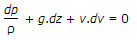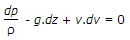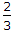# Mechanical Engineering - Hydraulics and Fluid Mechanics

### Exercise :: Hydraulics and Fluid Mechanics - Section 1

16.

According to equation of continuity,

 A. w1a1 = w2a2 B. w1v1 = w2v2 C. a1v1 = a2v2 D. a1/v1 = a2/v2

Explanation:

No answer description available for this question. Let us discuss.

17.

Coefficient of resistance is the ratio of

 A. actual velocity of jet at vena contracta to the theoretical velocity B. area of jet at vena contracta to the area of orifice C. loss of head in the orifice to the head of water available at the exit of the orifice D. actual discharge through an orifice to the dieoretical discharge

Explanation:

No answer description available for this question. Let us discuss.

18.

Euler's equation in the differential form for the motion of liquids is given by

 A.B.C. ρ.dp + g.dz + v.dv = 0 D. ρ.dp - g.dz + v.dv = 0

Explanation:

No answer description available for this question. Let us discuss.

19.

In order to measure the flow with a venturimeter, it is installed in

 A. horizontal line B. inclined line with flow upwards C. inclined line with flow downwards D. any direction and in any location

Explanation:

No answer description available for this question. Let us discuss.

20.

The discharge through a large rectangular orifice is given by (where H1 = Height of the liquid above the top of the orifice, H2 = Height of the liquid above the bottom of the orifice, b = Breadth of the orifice, and Cd = Coefficient of discharge)

 A. Q =Cd x b2g(H2 - H1) B. Q =Cd x b2g(H21/2 - H11/2) C. Q =Cd x b2g(H23/2 - H13/2) D. Q =Cd x b2g(H22 - H12)